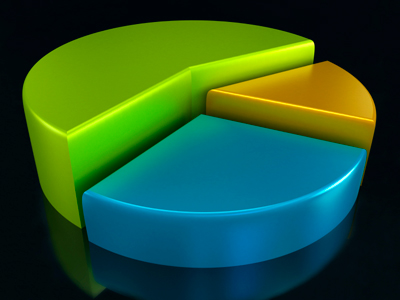Formulae can create output as charts and graphs like a pie chart.

# Models 2

This Computer Science quiz is called 'Models 2' and it has been written by teachers to help you if you are studying the subject at middle school. Playing educational quizzes is a fabulous way to learn if you are in the 6th, 7th or 8th grade - aged 11 to 14.

It costs only \$12.50 per month to play this quiz and over 3,500 others that help you with your school work. You can subscribe on the page at Join Us

You will often see formulae in computer models. Spreadsheets are frequently used for financial information because of their ability to re-calculate the entire sheet automatically after a change to a single cell is made.

Try our second quiz on Models.

1.
Which of these is not a formula?
=C3+F2*H5
=C3-F2*\$H5
-C3/F2*H5
=C3*F2*H5
2.
Models represent real life situations using .......
data and analysis
data and intuition
data and rules
data and statistics
3.
Spreadsheet models use ....... to represent rules.
cells
conditional formatting
formulae
symbols
4.
The values in the spreadsheet cells act as .......
information
input
macros
reports
5.
The formulae in the models act as .......
calculations
data
enquiries
processes
6.
The formulae can create output as charts and graphs.
Charts and graphs are the only form of output possible
Charts and graphs are vital to understand predictions
Charts and graphs can improve understanding of results
Charts and graphs make the output more accurate
Use them when they are useful - not just for looks
7.
Spreadsheets are good at doing ....... analysis of data.
how-why
what-else
what-if
why-when
For example: What happens to sales if tax rates increase?
8.
A model rule is: profit = number sold x saleprice - cost.
A suitable formula might be: =D5x(C5-B5)
A suitable formula might be: =D5x(C5/B5)
A suitable formula might be: =D5*(C5-B5)
A suitable formula might be: =D5^(C5-B5)
The 'rule' is represented by a formula entered in a cell
9.
Models often include many rules because .......
a single formula can only handle simple rules
real world situations are often very complicated
results can be achieved much quicker with more rules
the more rules used, the more accurate the results
Even simple situations can need many rules to model
10.
Predictions made by models are usually .......
approximate
correct
exact
wrong
Rules are rarely perfect and may not have complete data
Author:  Duncan Daish